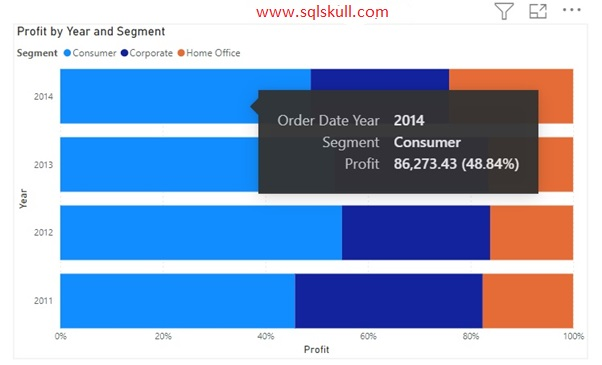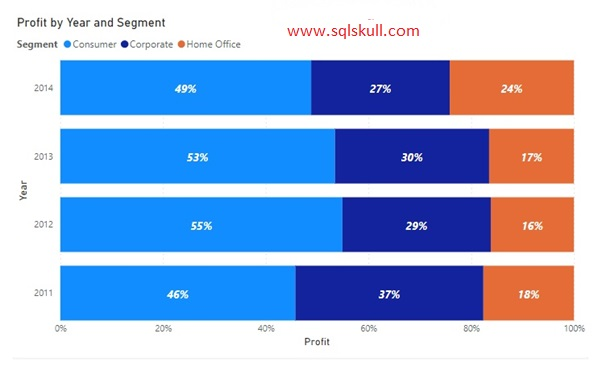Home » 100% Stacked bar chart in Power BI

# 100% Stacked bar chart in Power BIPower BI 100% stacked bar chart is used to display relative percentage of multiple data series in stacked bars, where the total (cumulative) of each stacked bar always equals 100%.
In a 100% stacked bar chart, Axis is represented on Y-axis and Value is represented on X-axis.

Lets see how to implement 100% stacked bar chart in Power BI.

In visualization pane, select the 100% stacked bar chart as shown below.Now, you you need to specify the following fields to create a 100% stacked bar chart.

• Axis: specify the field that represents the Horizontal Bars.
• Legend: specify the field to divide the Horizontal Bars.
• Values: specify the value such as total sales, or profit etc.

Lets, select the Order date- year field from fields pane and drag to Axis field.

Then, select the Profit field from fields pane and drag to Values field.

After that, select the Segment field from fields pane and drag to Legend field.

Once you done with this, you can see 100% stacked bar chart is created as shown below.When you hover over any portion of a bar, you will see a tool-tip displays an information of Segment, Order date- year and Profit( in percentage) of the total profit.Lets enable the data labels, for this go to the Format tab and enable the Data labels option as shown below.Once you enable the data labels, you can see the percentage values for each segment.You can also customize the percentage format, you can round the percentage to zero decimal places, or can increase the decimal places as shown below.

Lets remove the decimal place from percentage.

Go to format tab, under Data labels, go to Values and then type 0 in Value decimal place.